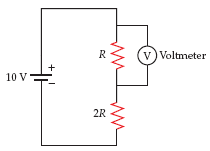# Potential difference across a resistor as measured by a voltmeter.

#### kballerina

1. The problem statement, all variables and given/known data

The voltmeter shown in the figure below can be modeled as an ideal voltmeter (a voltmeter that has an infinite internal resistance) in parallel with a 11.0-MΩ resistor. Calculate the reading on the voltmeter for the following.R = 1.1 MΩ

R = 10.8 MΩ

R = 104 MΩ

2. Relevant equations

V=IR
Rtotal=R+2R (since they are in series)

3. The attempt at a solution

My guess is that I am having two issues with this problem, the first being the problem statement itself, it gives me a value for a R and then tells me to solve the problem for the given values of R. I got the first two parts of the problem ( R = 0.9 kΩ and R = 9.7 kΩ both having the potential difference of 3.333 volts) To do those I divided the EMF by Rtotal (since the resistors are in series) to get the current then multiplied by the resistance of R. This worked fine for the first two but on the third one and beyond my problem arose and my answer was wrong. I'm not entirely sure why and the explanation in my book is quite limited so I'm having trouble finding my error on these last 3 parts of the problem. For all of my equations the answer was same potential difference (3.333 V)

Last edited:

#### rl.bhat

Homework Helper
Hi kballerina, welcome to PF.

Reading in the voltmeter is given by

V = EMF*R/3R.

And it is true for all the three cases.

#### willem2

Hi kballerina, welcome to PF.

Reading in the voltmeter is given by

V = EMF*R/3R.

And it is true for all the three cases.
You're forgetting the 11 M$$\Omega$$ internal resistance of the voltmeter.
It's parallel with resistance R.

### The Physics Forums Way

We Value Quality
• Topics based on mainstream science
• Proper English grammar and spelling
We Value Civility
• Positive and compassionate attitudes
• Patience while debating
We Value Productivity
• Disciplined to remain on-topic
• Recognition of own weaknesses
• Solo and co-op problem solving